## How to Calculate and Solve for the Length of a Side and Perimeter of a Cube | The Calculator Encyclopedia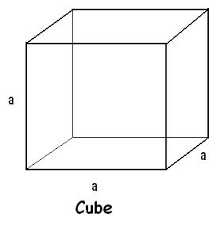The image above is a cube.

To compute the perimeter of a cube, one essential parameter is needed and this parameter is length of one of the sides (a).

The formula for calculating the perimeter of a cube:

P = 12a

Where;
P = Perimeter of the cube
a = Length of one of the sides of the cube

Let’s solve an example;
Find the perimeter of a cube when the length of one of the sides is 14 cm.

This implies that;
a = Length of one of the sides = 14 cm

P = 12a
P = 12 x 14
P = 168

Therefore, the perimeter of the cube is 168 cm.

Calculating the Length of one of the sides of a Cube using the Perimeter of the Cube.

a = P / 12

Where;
a = Length of one of the sides of the cube
P = Perimeter of the cube

Let’s solve an example;
Find the length of one of the sides of a cube when the perimeter of the cube is 200 cm.

This implies that;
P = Perimeter of the cube = 200 cm

a = P / 12
a = 200 / 12
a = 16.67

Therefore, the length of one of the sides is 16.67 cm.

## How to Calculate and Solve for the Perimeter of an Ellipse | Nickzom Calculator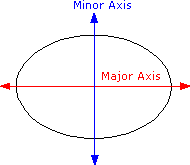The image above is an ellipse.

To compute the perimeter of an ellipse, two essential parameters are needed and this parameters are axis (a) and axis (b).

The formula for calculating the perimeter of an ellipse:

P = π [3(a + b) – √((3a + b)(a + 3b))]

Where;

P = Perimeter of the ellipse
a = Axis of the ellipse
b = Axis of the ellipse

Let’s solve an example;
Given that the axis of the ellipse (a) is 19 cm and axis of the ellipse (b) is 31 cm. Find the perimeter of the ellipse?

This implies that;
a = Axis of the ellipse = 19 cm
b = Axis of the ellipse = 31 cm

P = π [3(a + b) – √((3a + b)(a + 3b))]
P = 3.142 [3(19 + 31) – √((3 x 19 + 31)(19 + 3 x 31))]
P = 3.142 [3(50) – √((57+ 31)(19 + 93))]
P = 3.142 [150 – √((88)(112))]
P = 3.142 [150 – √(9856)]
P = 3.142 [150 – 99.28]
P = 3.142 [50.72]
P = 3.142 x 50.72
P = 159.26

Therefore, the perimeter of the ellipse is 159.26 cm.

## How to Calculate and Solve for the Length of a Side and Perimeter of a Hexagon | The Calculator EncyclopediaThe image above is a hexagon.

To compute the perimeter of a hexagon, one essential parameter is needed and this parameter is length of side (a).

The formula for calculating the perimeter of a pentagon:

P = 6a

Where;
P = Perimeter of the hexagon
a = length of side

Let’s solve an example;
Find the perimeter of a hexagon when the length of side is 15 cm.

This implies that;
a = length of side = 15 cm

P = 6a
P = 6 x 15
P = 90

Therefore, the perimeter of the hexagon is 90 cm.

Calculating the length of side (a) using the Perimeter of the hexagon.

a = P / 6

Where;
a = length of side
P = Perimeter of the hexagon

Let’s solve an example;
Find the length of side when the perimeter of the hexagon is 120 cm.

This implies that;
P = Perimeter of the hexagon = 120 cm

a = P / 6
a = 120 / 6
a = 20

Therefore, the length of side (a) is 20 cm.

## How to Calculate and Solve for the Length of a Side and Perimeter of a Pentagon | Nickzom Calculator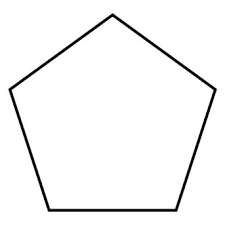The image above is a pentagon.

To compute the perimeter of a pentagon, one essential parameter is needed and this parameter is length of side (a).

The formula for calculating the perimeter of a pentagon:

P = 5a

Where;
P = Perimeter of the pentagon
a = length of side

Let’s solve an example;
Find the perimeter of a pentagon when the length of side (a) is 25 cm.

This implies that;
a = length of side = 25 cm

P = 5a
P = 5 x 25
P = 125

Therefore, the perimeter of the pentagon is 125 cm.

Calculating the length of side using the perimeter of the pentagon.

a = P / 5

Where;
a = Length of side
P = Perimeter of the pentagon

Let’s solve an example;
Given that the area of the pentagon is 250 cm. Find the length of side?

This implies that;
P = Perimeter of the pentagon = 250 cm

a = P / 5
a = 250 / 5
a = 50

Therefore, the length of side (a) is 50 cm.

## How to Calculate and Solve for the Length and Perimeter of a Rhombus | Nickzom Calculator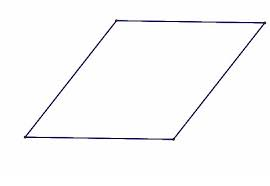The image above is a rhombus.

To compute the area of a rhombus, one essential parameter is needed and this parameter is length of side (a).

The formula for calculating the perimeter of a rhombus:

P = 4a

Where;
P = Perimeter of the rhombus
a = length of side

Let’s solve an example;
Find the perimeter of a rhombus when the length of side is 30 cm.

This implies that;
a = Length of side = 30 cm

P = 4a
P = 4 x 30
P = 120

Therefore, the perimeter of the rhombus is 120 cm.

Calculating the Length of Side using the Perimeter of the Rhombus.

a = P / 4

Where;
a = Length of Side
P = Perimeter of the rhombus

Let’s solve an example;
Given that the perimeter of the rhombus is 180 cm. Find the length of side?

a = P / 4

This implies that;
P = perimeter of the rhombus = 180 cm

a = P / 4
a = 180 / 4
a = 45

Therefore, the length of side is 45 cm.

## How to Calculate and Solve for the Perimeter of a Trapezium | Nickzom Calculator

The image above is a trapezium.

To compute the perimeter of a trapezium, four essential parameters is needed and they are the length of the side (a), length of the side (b), length of the side (c) and length of the side (d).

The formula for calculating the perimeter of a trapezium is;

P = a + b + c + d

Where;

P = Perimeter of the trapezium
a = length of the side
b = length of the side
c = length of the side
d = length of the side

Let’s solve an example;
Find the perimeter of a trapezium with length of side (a) 4 cm, length of side (b) 8 cm, length of side (c) 12, length of side (d) 17 cm.

This implies that;
a = length of side = 4 cm
b = length of side = 8 cm
c = length of side = 12 cm
d = length of side = 17 cm

P = a + b + c + d
P = 4 + 8 + 12 + 17
P = 41

Therefore, the perimeter of the trapezium is 41 cm.

## How to Calculate and Solve for the Perimeter or Circumference, Diameter, Radius and Angle of a Sector | The Calculator Encyclopedia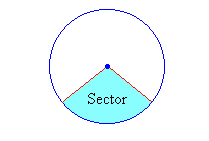The image above is a sector.

To compute the Perimeter or Circumference a sector, two essential parameters is needed and this parameters are the radius of the sector (r) and the angle of the sector (θ). You can also use the diameter of the sector (d).

The formula for calculating the Perimeter or Circumference of a sector:

P = 2r + θ / 360(2πr)

Where;

P = Perimeter or Circumference
r = Radius of the sector
θ = Angle of the Sector

Let’s solve an example:
Find the perimeter or circumference of a sector when the radius of the sector is 14 cm and the angle of the sector is 60°

This implies that;

r = Radius of the sector = 14 cm
θ = Angle of the sector = 60°

P = 2r + θ / 360(2πr)
P = 2 x 14 + 60 / 360(2 x 3.142 x 14)
P = 28 + 0.1667 (87.97)
P = 28 + 14.66
P = 42.66

Therefore, the perimeter or circumference of the sector is 42.66 cm.

Calculating the Perimeter or Circumference of a Sector using Diameter and Angle of the sector.

P = d + θ / 360(πd)

θ = Angle of the sector
d = Diameter of the sector

Let’s solve an example;
Find the perimeter or circumference of a sector when the diameter of the sector is 20 cm and the angle of the sector is 80°.

This implies that;

d = Diameter of the sector = 20 cm
θ = Angle of the sector = 80°

## How to Calculate and Solve for the Perimeter or Circumference, Radius and Diameter of a Circle | The Calculator Encyclopedia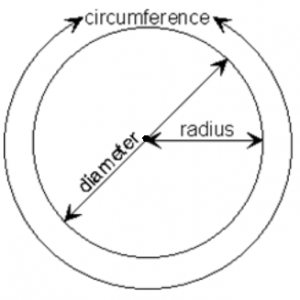The image above is a circle.

To compute the perimeter or circumference of a circle, one essential parameter is needed and this parameter is the radius of a circle (r). You can also use the diameter of a circle to compute the area of a circle (d).

The formula for calculating the perimeter or circumference of a circle is:

P = 2πr

Where:

P = Perimeter or Circumference of a circle
r = Radius of a circle

Let’s solve an example:
Find the perimeter or circumference of a circle where the radius of a circle is 8 cm.

This implies that;
r = Radius of a circle = 8 cm

P = 2πr
P = 2 x 3.142 x 8
P = 50.265

Therefore, the perimeter or circumference of a circle is 50.265 cm.

Calculating the Area of a Circle using the Diameter of a Circle.

The formula is P = πd

Where:

P = Perimeter or Circumference of a circle
d = Diameter of a circle

Let’s solve an example:
Find the perimeter or circumference of a circle where the diameter of a circle is 10 cm.

This implies that;
d = Diameter of a circle = 10 cm

P = πd
P = 3.142 x 10
P = 31.42

Therefore, the perimeter or circumference of a circle with diameter is 31.42 cm.

How to Calculate Radius of a Circle when Perimeter or Circumference of the Circle is Given

r = P /

where;

r = Radius of a circle
P = Perimeter or Circumference of a circle

Let’s solve an example:
Find the radius of a circle where the perimeter or circumference of the circle is 16 cm.

This implies that;
P = Perimeter or Circumference of the circle = 16 cm

r = P /
r = 16 / 6.284
r = 2.55

Therefore, the radius of the circle is 2.55 cm.

How to Calculate Diameter of a Circle when Perimeter or Circumference of the Circle is Given

d = P / π

where;

d = Diameter of a circle
P = Perimeter or Circumference of a circle

Let’s solve an example;
Find the diameter of a circle where the perimeter or circumference of the circle is 20 cm

This implies that;
P = Perimeter or Circumference of the circle = 20 cm

d = P / π
d = 20 / π
d = 6.365

Therefore, the diameter of the circle is 6.365 cm.

## How to Calculate and Solve for the Perimeter, Length and Width of a Rectangle | The Calculator EncyclopediaThe image above is a rectangle with a length of 8 cm and a width of 5 cm.
To compute the perimeter of a rectangle requires two parameters which are the length of the rectangle and the width of the rectangle.

The formula for calculating the perimeter of a rectangle is:

P = 2 (l + w)

Where:

P = Perimeter of a Rectangle
l = Length of a Rectangle
w = Width of a Rectangle

Let’s solve an example:
Find the perimeter of a rectangle where the length of the rectangle is 8 cm and the width of the rectangle is 5 cm.

This implies that:
l = Length of the Rectangle = 8 cm
w = Width of the Rectangle = 5 cm

P = 2 (l + w)
P = 2 (8 + 5)
P = 2 (13)
P = 26

Therefore, the perimeter of the rectangle is 26 cm.

How to Calculate the Length of a Rectangle when the Perimeter of the Rectangle and the Width of the Rectangle is Given

l = P / 2 – w

Where:
l = Length of the Rectangle
P = Perimeter of the Rectangle
w = Width of the Rectangle

## How to Calculate and Solve for the Perimeter and Length of a Square | The Calculator Encyclopedia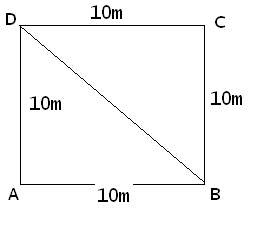The image above represents a square.
To compute the perimeter of a square requires one essential parameter which is the length of one side of a square.

The formula for computing the perimeter of a square is:

P = 4 x l

Where:
P = Perimeter of a Square
l = Length of one side of a Square

Let’s solve an example
Find the perimeter of a square which has a length of 10 m.

This implies that:
l = length of one side of a square = 10

P = 4 x l
P = 4 x 10
P = 40

Therefore, the perimeter of the square is 40 m.

Calculating the Length of a Square when Perimeter is Given

The formula is l = p / 4

Where;
P = Perimeter of a square
l = Length of a square

Let’s solve an example:
Find the length of a square where the perimeter of a square is 100 m.

This implies that;
P = Perimeter of a square = 100 m

l = p / 4
l = 100 / 4
l = 25

Therefore, the length of the square is 25 m.